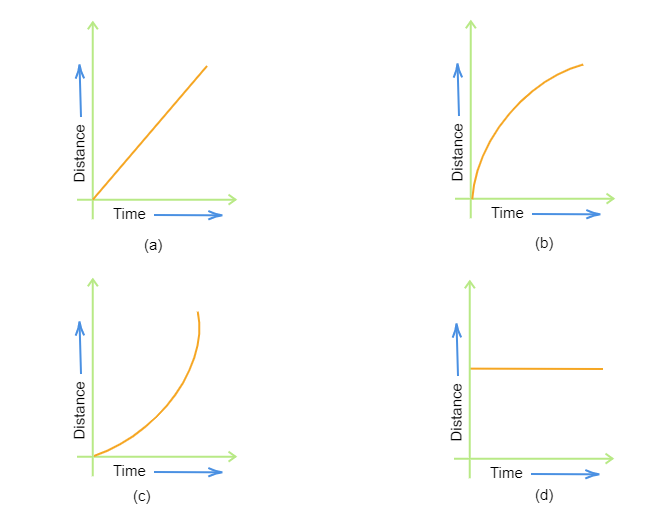# Which of the following figures represents the uniform motion of a moving object correctly?"

When a moving object covers equal distance in equal time intervals, then its speed is said to be moving in a uniform motion.

In the given graphs, only graph $(a)$ indicates uniform motion.

So, option $(a)$ is correct.